# Torsion tensor derivation

• A
mertcan
hi, I looked up torsion tensor derivation on 2 different books, and encountered 2 different situations, so my mind has been confused. For the first image, I could totally understand how torsion tensor was derived, but for the second image although there are similar things, I can not make a connection between 2 images. For instance, in first image torsion tensor is between r1 and r2 points, but this torsion tensor does not correspond to torsion tensor in second image, because instead there is a [u,v] vector. Besides, as you can see "sr1" is the parallel transported vector according to first image and resembles the vector "uR" ( appearance of these 2 vectors is same I think), but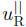is parallel transported vector according to second image ( it is really parallel to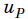vector, but for the first image the parallel transported vector ("sr1" ) is not really parallel to " pq " vector. To sum up, Could you please help me understand these 2 different situation, and express or explain the truth?

FIRST IMAGE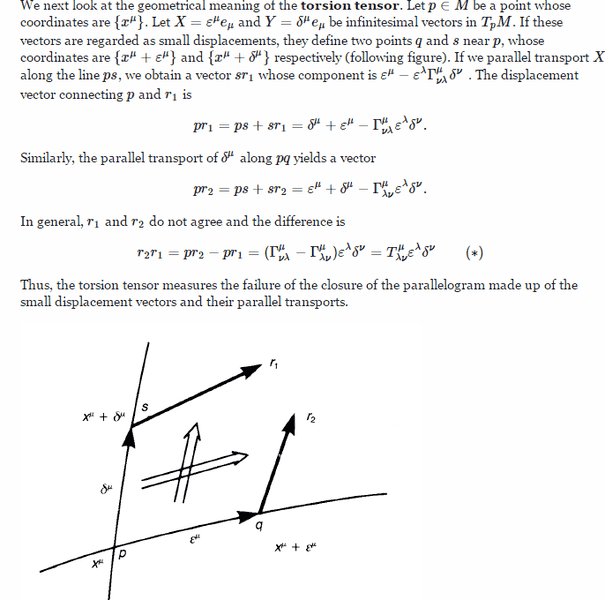SECOND IMAGE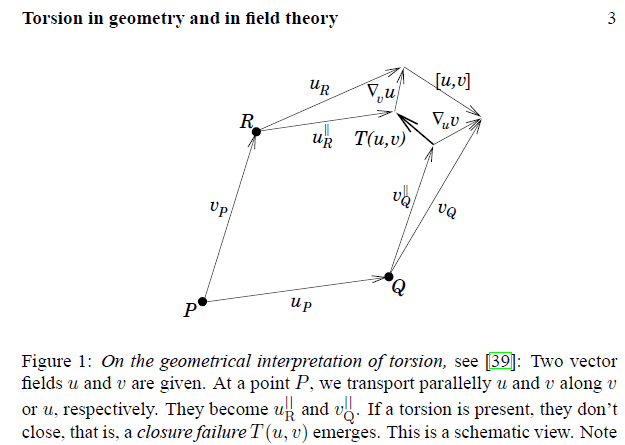mertcan
hi, I looked up torsion tensor derivation on 2 different books, and encountered 2 different situations, so my mind has been confused. For the first image, I could totally understand how torsion tensor was derived, but for the second image although there are similar things, I can not make a connection between 2 images. For instance, in first image torsion tensor is between r1 and r2 points, but this torsion tensor does not correspond to torsion tensor in second image, because instead there is a [u,v] vector. Besides, as you can see "sr1" is the parallel transported vector according to first image and resembles the vector "uR" ( appearance of these 2 vectors is same I think), but View attachment 101110 is parallel transported vector according to second image ( it is really parallel to View attachment 101111 vector, but for the first image the parallel transported vector ("sr1" ) is not really parallel to " pq " vector. To sum up, Could you please help me understand these 2 different situation, and express or explain the truth?

FIRST IMAGE

View attachment 101107

SECOND IMAGE

View attachment 101108

Why anyone can help me?? Is it a though question or long question ??

mertcan
hi, I looked up torsion tensor derivation on 2 different books, and encountered 2 different situations, so my mind has been confused. For the first image, I could totally understand how torsion tensor was derived, but for the second image although there are similar things, I can not make a connection between 2 images. For instance, in first image torsion tensor is between r1 and r2 points, but this torsion tensor does not correspond to torsion tensor in second image, because instead there is a [u,v] vector. Besides, as you can see "sr1" is the parallel transported vector according to first image and resembles the vector "uR" ( appearance of these 2 vectors is same I think), but View attachment 101110 is parallel transported vector according to second image ( it is really parallel to View attachment 101111 vector, but for the first image the parallel transported vector ("sr1" ) is not really parallel to " pq " vector. To sum up, Could you please help me understand these 2 different situation, and express or explain the truth?

FIRST IMAGE

View attachment 101107

SECOND IMAGE

View attachment 101108
hi, I looked up torsion tensor derivation on 2 different books, and encountered 2 different situations, so my mind has been confused. For the first image, I could totally understand how torsion tensor was derived, but for the second image although there are similar things, I can not make a connection between 2 images. For instance, in first image torsion tensor is between r1 and r2 points, but this torsion tensor does not correspond to torsion tensor in second image, because instead there is a [u,v] vector. Besides, as you can see "sr1" is the parallel transported vector according to first image and resembles the vector "uR" ( appearance of these 2 vectors is same I think), but View attachment 101110 is parallel transported vector according to second image ( it is really parallel to View attachment 101111 vector, but for the first image the parallel transported vector ("sr1" ) is not really parallel to " pq " vector. To sum up, Could you please help me understand these 2 different situation, and express or explain the truth?

FIRST IMAGE

View attachment 101107

SECOND IMAGE

View attachment 101108

Why can anyone help me?? Is it a though question or long ??

Markus Hanke
I don't really understand your question, I'm afraid; the two diagrams depict the exact same thing, the only difference is that the various vectors have different labels. In both cases, torsion is demonstrated as being the failure of the parallelogram to be closed.

2022 Award
I think all this is correct, but do be a bit wary. Still learning this myself.

The second image is comparing and contrasting two similar definitions: "You draw a parallelogram and the failure to close is the torsion" and "You draw a parallelogram and the failure to close is the commutator". The inner parallelogram is the same as your first diagram. The outer parallelogram is something different but confusingly similar.

The inner parallelogram's top and right sides are formed by parallel transporting the bottom and left sides along the left and bottom sides respectively - the failure to close is the torsion. The outer parallelogram's top and right sides are the values of the vector field at R and Q respectively - the failure to close is the commutator.

The difference between the two top sides (i.e. the difference between the parallel transported vector and the "local" value) is the covariant derivative in the direction of the left side. Similarly for the two right sides.

mertcan
Markus Hanke they do not depict the same thing, look at the parallel transported vectors. The parallel transported vector "sr1" in first image should correspond to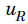vector notvector in the second image. By the way Ibix, how are these 2 different derivations correct ?? I mean torsion definition must be unique, but there are 2 different meanings for torsion in these images. How can we have 2 different torsion definition ? If we say that torsion definition is the the failure of the closure of the parallelogram made up of the small displacement vectors and their parallel transports, we can not use that definition for second image, because in second image we should not haveor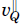vectors as a parallel transported vectors

mertcan
Markus Hanke they do not depict the same thing, look at the parallel transported vectors. The parallel transported vector "sr1" in first image should correspond to View attachment 101127 vector not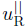vector in the second image. By the way Ibix, how are these 2 different derivations correct ?? I mean torsion definition must be unique, but there are 2 different meanings for torsion in these images. How can we have 2 different torsion definition ? If we say that torsion definition is the the failure of the closure of the parallelogram made up of the small displacement vectors and their parallel transports, we can not use that definition for second image, because in second image we should not haveor View attachment 101128 vectors as a parallel transported vectors
Guys, I have been waiting for a long time, and I have not received an answer. I really want to make you sure that I can not make any progress in this topic. I got stuck, and I want to proceed to other tremendous, astonishing topics in relativity. I will appreciate, and be so pleased if you return me...

Staff Emeritus
Homework Helper
Gold Member
Markus Hanke they do not depict the same thing, look at the parallel transported vectors. The parallel transported vector "sr1" in first image should correspond to View attachment 101127 vector notvector in the second image.

No, this is incorrect, sr1 corresponds to ##u_R^\parallel##. The vectors ##u_R##/##v_Q## are not parallel transported along the parallelogram sides.

•haushofer
mertcan
No, this is incorrect, sr1 corresponds to ##u_R^\parallel##. The vectors ##u_R##/##v_Q## are not parallel transported along the parallelogram sides.
Thank you for your return "Orodruin" ,but if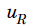or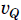are not parallel transported vectors, for what do they stand? also What is the meaning ofandvector ?

Staff Emeritus
Homework Helper
Gold Member
The first one seems to come from Nakahara.

mertcan
The vector fields at the specific points.

Transporting a vector field from some point to a point Q is different from simply evaluating it in point Q. Is that your confusion?
Thank you for your informing "Orodruin". As " haushofer" guessed, first image belongs to "Nakahara", whereas second image belongs to "F.W. Hehl and Yu.N. Obukhov". I consider the problem is: There may be a parallel transportation problem of vectors. For example, parallel transported vector "sr1" in first image do not parallel to "pq" vector, but if we pass to the second image we can examine that parallel transported vectoris totally parallel to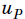vector, and this situation makes a confusion. Nevertheless, "Orodruin" expressed that sr1 corresponds to. The vectors, and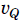are not parallel transported along the parallelogram sides. After this explanation I have one more question: iforare not parallel transported vectors, for what do they stand? also What is the meaning ofandvector ?

Staff Emeritus
Homework Helper
Gold Member
Thank you for your informing "Orodruin". As " haushofer" guessed, first image belongs to "Nakahara", whereas second image belongs to "F.W. Hehl and Yu.N. Obukhov". I consider the problem is: There may be a parallel transportation problem of vectors. For example, parallel transported vector "sr1" in first image do not parallel to "pq" vector, but if we pass to the second image we can examine that parallel transported vectoris totally parallel to View attachment 101142 vector, and this situation makes a confusion. Nevertheless, "Orodruin" expressed that sr1 corresponds to. The vectors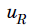, andare not parallel transported along the parallelogram sides. After this explanation I have one more question: iforare not parallel transported vectors, for what do they stand? also What is the meaning ofandvector ?

•haushofer
I have a hard time guessing what the problem is, since we have answered your questions already. Why don't you agree with post #8?

mertcan
hi, I have been digging up torsion tensor, and I would like to share 2 different images below. At the first image, I suppose that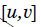is the difference of directional derivatives, because at the second image this type of representation corresponds to difference of directional derivatives. If we come to the first image we can say that=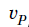+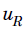-(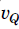+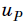), but we are aware that as I said beforeis the difference of directional derivatives according to the second image. To sum up, in accordance with the=+-(+) formula,+should be a directional derivative, but in line with the second image directional derivative is not necessarily tangent to manifold, as you can see first image totally lays on the tangent place,+is tangent to manifold instead of being not tangent according to second image.

First image is quoted from F.W. Hehl and Yu.N. Obukhov
second image quoted from David R. Wilkins

FIRST IMAGE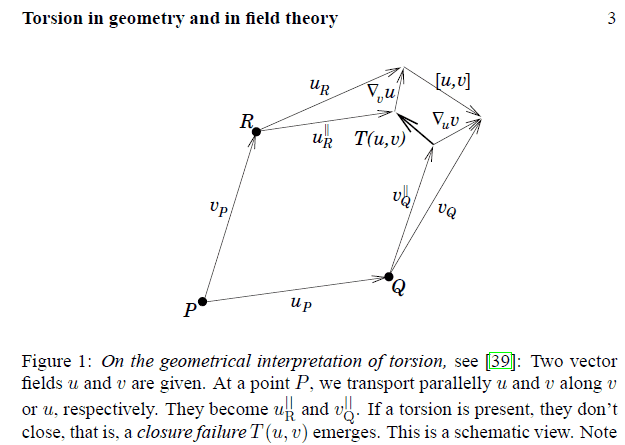SECOND IMAGE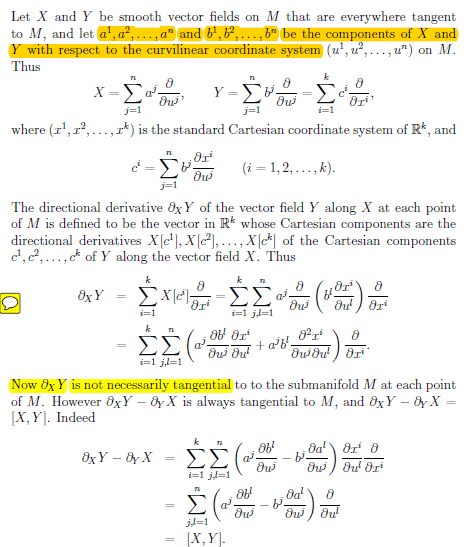Staff Emeritus
Homework Helper
Gold Member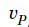+should be a directional derivative
No, it should not. These are two completely different vectors which a priori has nothing to do with each other.

Also, please use the LaTeX features of the forum rather than editing in images, it will make your posts significantly more readable.

mertcan
No, it should not. These are two completely different vectors which a priori has nothing to do with each other.

Also, please use the LaTeX features of the forum rather than editing in images, it will make your posts significantly more readable.
Well thanks for your return, but why do we put this [u,v] vector (at the second image ) on the image?? How we can derive this vector?? The only derivation I know of this vector is the difference of directional derivatives, but I can not see any directional derivatives ( after " Orodruin" 's explanation ) at the first image. What kind of logic exist for that vector??

Staff Emeritus
Homework Helper
Gold Member
##[u,v]## is just the failure of the flows of the vector fields ##u## and ##v## to close. In general, I suggest that you do not try to see properties of a manifold in terms of an embedding as is done in your second image in #16. The properties under discussion are perfectly well defined only in terms of the properties of the manifold and affine connection itself (even without the affine connection when we just discuss the Lie bracket) without reference to an embedding in a higher-dimensional space.

mertcan
##[u,v]## is just the failure of the flows of the vector fields ##u## and ##v## to close. In general, I suggest that you do not try to see properties of a manifold in terms of an embedding as is done in your second image in #16. The properties under discussion are perfectly well defined only in terms of the properties of the manifold and affine connection itself (even without the affine connection when we just discuss the Lie bracket) without reference to an embedding in a higher-dimensional space.
##[u,v]## is just the failure of the flows of the vector fields ##u## and ##v## to close. In general, I suggest that you do not try to see properties of a manifold in terms of an embedding as is done in your second image in #16. The properties under discussion are perfectly well defined only in terms of the properties of the manifold and affine connection itself (even without the affine connection when we just discuss the Lie bracket) without reference to an embedding in a higher-dimensional space.
Do you mean that [u,v] is not the difference of directional derivatives?? If not, how do we calculate the value of it??

Staff Emeritus
Homework Helper
Gold Member
Do you mean that [u,v] is not the difference of directional derivatives?? If not, how do we calculate the value of it??
If you use "directional derivative" to mean "as seen embedded into a Cartesian space" then no. The Lie bracket ##[u,v]## is the commutator between the vector fields ##u## and ##v##, which you can easily show is a vector field in itself. You can easily show that it is related to the failure of flows to commute.

mertcan
If you use "directional derivative" to mean "as seen embedded into a Cartesian space" then no. The Lie bracket ##[u,v]## is the commutator between the vector fields ##u## and ##v##, which you can easily show is a vector field in itself. You can easily show that it is related to the failure of flows to commute.
Well, Could you give some specific examples or remind me how to calculate the [u,v] commutator. For instance, If I want to find a torsion tensor, I need covariant derivatives, and [u,v] commutator, but I know the formula of how to calculate the covariant derivative whereas I can not remember the formulation of commutator. What kind of proof may make a relation between the failure of flows and commutator?? I am asking because I want to visualize the concept using some proofs.

Staff Emeritus
Well, Could you give some specific examples or remind me how to calculate the [u,v] commutator. For instance, If I want to find a torsion tensor, I need covariant derivatives, and [u,v] commutator, but I know the formula of how to calculate the covariant derivative whereas I can not remember the formulation of commutator. What kind of proof may make a relation between the failure of flows and commutator?? I am asking because I want to visualize the concept using some proofs.

I hope I don't screw this up, but here's my understanding:

If you have a scalar function $\phi$, and a tangent vector $u$, then the meaning of $u(\phi)$ is the rate of change of $\phi$ along the tangent $u$. In terms of coordinates, it's $u(\phi) = \sum_j u^j \frac{\partial \phi}{\partial x^j}$.

The meaning of $[u,v]$ is that tangent vector $w$ such that $w(\phi) = u(v(\phi)) - v(u(\phi))$

In terms of coordinates, $[u,v] = w \Rightarrow w^j = \sum_i (u^i \frac{\partial v^j}{\partial x^i} - v^i \frac{\partial u^j}{\partial x^i})$

Staff Emeritus
In terms of coordinates, $[u,v] = w \Rightarrow w^j = \sum_i (u^i \frac{\partial v^j}{\partial x^i} - v^i \frac{\partial u^j}{\partial x^i})$

Just for clarification of what I wrote, the commutator $[u,v]$ only makes sense if $u$ and $v$ are vector fields, not vectors. A vector field assigns a vector to each point in your space. In terms of components, $u^i$ is a function of position.

mertcan
Just for clarification of what I wrote, the commutator $[u,v]$ only makes sense if $u$ and $v$ are vector fields, not vectors. A vector field assigns a vector to each point in your space. In terms of components, $u^i$ is a function of position.
Ok, I got your answer, but could you show me at the image where u(v(ϕ)) and v(u(ϕ)) take place? I am asking because without seeing u(v(ϕ)) and v(u(ϕ)) vector, it seems to me as if [u,v] vector was conjured up...Staff Emeritus
Let me first show how it works in the good-old 2D Euclidean plane. Since that space is flat, you can choose Cartesian coordinates, and you don't have to worry about connection coefficients.

Now, suppose we have two different vector fields, $\vec{u}$ and $\vec{v}$. Then consider two different paths starting from the point $p_0$
1. Move along displacement vector $\alpha \vec{u}$, and then move along displacement vector $\beta \vec{v}$.
2. Move along displacement vector $\beta \vec{v}$, and then move along displacement vector $\alpha \vec{u}$.
As shown in the attachment, path 1 consists of going from $p_0$ to $p_1$ to $p_2$. Path 2 consists of going from $p_0$ to $p_3$ to $p_4$

In general, $p_2 \neq p_4$. We can compute, to lowest order, these two locations, as follows:

$p_1 = p_0 + \beta\vec{v}(p_0)$
$p_2 = p_1 + \alpha \vec{u}(p_1) \approx p_0 + \beta\vec{v}(p_0) + \alpha\vec{u}(p_0) + \alpha \beta ((\vec{v} \cdot \nabla) \vec{u})|_{p_0}$
$p_3 = p_0 + \alpha \vec{u}(p_0)$
$p_4 = p_3 + \beta \vec{v}(p_3) \approx p_0 + \beta\vec{v}(p_0) + \alpha \vec{u}(p_0) + \alpha \beta ((\vec{u} \cdot \nabla) \vec{v})|_{p_0}$

So the displacement vector from $p_2$ to $p_4$ is given by:

$\vec{\delta_{24}} = p_4 - p_2 = \alpha \beta ((\vec{u} \cdot \nabla) \vec{v} - (\vec{v} \cdot \nabla) \vec{u})|_{p_0}$
$\equiv [u,v]|_{p_0}$

#### Attachments

Staff Emeritus
Let me first show how it works in the good-old 2D Euclidean plane. Since that space is flat, you can choose Cartesian coordinates, and you don't have to worry about connection coefficients.

Now, suppose we have two different vector fields, $\vec{u}$ and $\vec{v}$. Then consider two different paths starting from the point $p_0$
1. Move along displacement vector $\alpha \vec{u}$, and then move along displacement vector $\beta \vec{v}$.
2. Move along displacement vector $\beta \vec{v}$, and then move along displacement vector $\alpha \vec{u}$.
As shown in the attachment, path 1 consists of going from $p_0$ to $p_1$ to $p_2$. Path 2 consists of going from $p_0$ to $p_3$ to $p_4$

In general, $p_2 \neq p_4$. We can compute, to lowest order, these two locations, as follows:

$p_1 = p_0 + \beta\vec{v}(p_0)$
$p_2 = p_1 + \alpha \vec{u}(p_1) \approx p_0 + \beta\vec{v}(p_0) + \alpha\vec{u}(p_0) + \alpha \beta ((\vec{v} \cdot \nabla) \vec{u})|_{p_0}$
$p_3 = p_0 + \alpha \vec{u}(p_0)$
$p_4 = p_3 + \beta \vec{v}(p_3) \approx p_0 + \beta\vec{v}(p_0) + \alpha \vec{u}(p_0) + \alpha \beta ((\vec{u} \cdot \nabla) \vec{v})|_{p_0}$

So the displacement vector from $p_2$ to $p_4$ is given by:

$\vec{\delta_{24}} = p_4 - p_2 = \alpha \beta ((\vec{u} \cdot \nabla) \vec{v} - (\vec{v} \cdot \nabla) \vec{u})|_{p_0}$
$\equiv [u,v]|_{p_0}$

If you do the same thing with non-Cartesian coordinates in curved space, you get additional terms in $p_4 - p_2$ that are due to connection coefficients, in addition to $[u,v]$.

(Note: in curved space, you can't interpret a tangent vector as a "displacement", but you can sloppily think of it that way as long as the size of the parallelogram is small enough.)

Staff Emeritus
If you do the same thing with non-Cartesian coordinates in curved space, you get additional terms in $p_4 - p_2$ that are due to connection coefficients, in addition to $[u,v]$.

(Note: in curved space, you can't interpret a tangent vector as a "displacement", but you can sloppily think of it that way as long as the size of the parallelogram is small enough.)

In curved space,

$\nabla_u v = \nabla_u (\sum_j v^j e_j)$ where $e_j$ is a set of basis vectors. We can use the properties of derivatives to write:

$\nabla_u v = (\sum_j \nabla_u v^j) e_j + \sum_j v^j (\nabla_u e_j)$

Similarly, $\nabla_v u = (\sum_j \nabla_v u^j) e_j + \sum_j u^j (\nabla_v e_j)$

So the difference is:

$\nabla_u v - \nabla_v u = \sum_j (\nabla_u v^j - \nabla_v u^j) e_j + \sum_j (v^j \nabla_u e_j) - \sum_i (u^i \nabla_v e_i)$

We can identify the terms on the left:
$\sum_j (\nabla_u v^j - \nabla_v u^j) e_j \equiv [u,v]$

$\sum_j (v^j \nabla_u e_j) \equiv \sum_{ijk} u^i v^j \Gamma^k_{ij} e_k$
$\sum_i (u^i \nabla_v e_i) \equiv \sum_{ijk} u^i v^j \Gamma^k_{ji} e_k$

So:
$\nabla_u v - \nabla_v u = [u,v] + \sum_{ijk} (u^i v^j (\Gamma^k_{ij} - \Gamma^k_{ji}))$

In a coordinate basis (which is what I'm assuming), $T(u,v) = \sum_{ijk} (u^i v^j (\Gamma^k_{ij} - \Gamma^k_{ji}))$, so we have:
$\nabla_u v - \nabla_v u = [u,v] + T(u,v)$

 That should be: $T(u,v) = \sum_{ijk} (u^i v^j (\Gamma^k_{ij} - \Gamma^k_{ji})e_k)$,

which is what is being shown pictorially in your diagram.

Last edited:
•Markus Hanke and vanhees71
mertcan
In curved space,

$\nabla_u v = \nabla_u (\sum_j v^j e_j)$ where $e_j$ is a set of basis vectors. We can use the properties of derivatives to write:

$\nabla_u v = (\sum_j \nabla_u v^j) e_j + \sum_j v^j (\nabla_u e_j)$

Similarly, $\nabla_v u = (\sum_j \nabla_v u^j) e_j + \sum_j u^j (\nabla_v e_j)$

So the difference is:

$\nabla_u v - \nabla_v u = \sum_j (\nabla_u v^j - \nabla_v u^j) e_j + \sum_j (v^j \nabla_u e_j) - \sum_i (u^i \nabla_v e_i)$

We can identify the terms on the left:
$\sum_j (\nabla_u v^j - \nabla_v u^j) e_j \equiv [u,v]$

$\sum_j (v^j \nabla_u e_j) \equiv \sum_{ijk} u^i v^j \Gamma^k_{ij} e_k$
$\sum_i (u^i \nabla_v e_i) \equiv \sum_{ijk} u^i v^j \Gamma^k_{ji} e_k$

So:
$\nabla_u v - \nabla_v u = [u,v] + \sum_{ijk} (u^i v^j (\Gamma^k_{ij} - \Gamma^k_{ji}))$

In a coordinate basis (which is what I'm assuming), $T(u,v) = \sum_{ijk} (u^i v^j (\Gamma^k_{ij} - \Gamma^k_{ji}))$, so we have:
$\nabla_u v - \nabla_v u = [u,v] + T(u,v)$

which is what is being shown pictorially in your diagram.
Initially, I really appreciate your effort, your endeavour "stevendarly"Your sharing is so valuable, remarkable, and significant in my opinion. To sum up, I would like to ask one more final question about the terminology of your sharing in Torsion tensor derivation: I can not catch the meaning of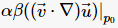entirely in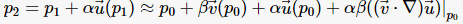( post#26). I have some thoughts as well as doubts about this situation, hence want to be sure: for what does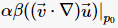stand? What is the aim and meaning of it??

Staff Emeritus
Initially, I really appreciate your effort, your endeavour "stevendarly"Your sharing is so valuable, remarkable, and significant in my opinion. To sum up, I would like to ask one more final question about the terminology of your sharing in Torsion tensor derivation: I can not catch the meaning of [deleted]

It's better if you used LaTex for formulas, instead of trying to get screenshots.

There is no point to $\alpha$ and $\beta$ other than that they are small positive real numbers, so that we can expand things in a power series in $\alpha$ and $\beta$ and just keep the lowest-order nonzero terms.

If $\vec{u}$ is a vector field, then that means that for each point $p$ in your space, then $\vec{u}(p)$ is a vector. In terms of coordinates (I'll assume $x,y, z$, then we have $\vec{u}(x,y,z)$.
• Let $p_0$ be some point with coordinates $x, y, z$.
• Let $v_0$ be the value of $v$ at that point. Let it have coordinates $v_0^x, v_0^y, v_0^z$
• Let $\beta$ be a small positive real number.
• Let $p_1$ be the point $p_0 + \beta \vec{v}$, that is, the point with coordinates $x + \beta v_0^x, y + \beta v_0^y, z + \beta v_0^z$
• Let $u_0$ be the value of $u$ at point $p_0$. Let it's coordinates be $u_0^x, u_0^y, u_0^z$
• Let $u_1$ be the value of $u$ at point $p_1$.
• Let $p_2$ be the point $p_1 + \alpha u_1$.
How do we calculate the components of $u_1$? Well, any smooth function of position can be expanded in a Taylor series:

$f(p_1) \approx f(p_0) + \frac{\partial f}{\partial x}|_{p_0} \delta x + \frac{\partial f}{\partial y}|_{p_0} \delta y + \frac{\partial f}{\partial z}|_{p_0} \delta z$, where $|_{p_0}$ means to evaluate the partial derivative at the point $p_0$ and where $\delta x$ means the change in $x$ in going from $p_0$ to $p_1$, and similarly for $\delta y$ and $\delta z$.

We can write that more compactly as $f(p_1) \approx f(p_0) + \sum_j \delta x^j \frac{\partial f}{\partial x^j}$, or even more compactly as:

$f(p_1) \approx f(p_0) + (\vec{\delta p} \cdot \nabla) f|_{p_0}$

where $\vec{\delta p} \cdot \nabla$ is the operator $\sum_j \delta x^j \frac{\partial}{\partial x^j}$ , and where $\delta p$ is the displacement vector from $p_0$ to $p_1$.

So in our case, we are interested in $u_1 \equiv u(p_1)$. So $u_1 \equiv u(p_1) = u(p_0) + (\vec{\delta p} \cdot \nabla) u|_{p_0}$. The displacement vector from $p_0$ to $p_1$ is just $\beta\vec{v}$, so we have:

$u_1 \equiv u(p_1) \approx u(p_0) + \beta (\vec{v} \cdot \nabla) u|_{p_0}$

Now, we define $p_2$ to be the point $p_1 + \alpha \vec{u_1} = p_0 + \beta \vec{v_0} + \alpha \vec{u_0} + \alpha \beta (\vec{v} \cdot \nabla) u|_{p_0}$

Last edited: